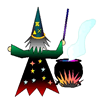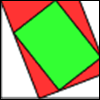#### You may also like### Where Can We Visit?

Charlie and Abi put a counter on 42. They wondered if they could visit all the other numbers on their 1-100 board, moving the counter using just these two operations: x2 and -5. What do you think?### The Mathemagician's Seven Spells

"Tell me the next two numbers in each of these seven minor spells", chanted the Mathemagician, "And the great spell will crumble away!" Can you help Anna and David break the spell?### Folding, Cutting and Punching

Exploring and predicting folding, cutting and punching holes and making spirals.

# Ishango Bone

##### Age 7 to 18Challenge Level

We received many interesting ideas concerning the Ishango bone. Thanks to you all for sending in your comments.

We were particularly pleased to hear from Huylebrouck at the Department of Archeology in Brussels, who gave us these fact concerning the bone:

A second Ishango rod was found indeed.

The Ishango rods are not "believed" to be 22000 years old. They are: they were dated through Carbon 14.

Alex and Theo said

We looked at Row C and found that $9 + 10=19$ and that $21 - 10 = 11$, the top line is all prime numbers so the bone could be a mathematical aid in the shape of a pen so people could use it as they worked.

Ben and Joshua from Lancaster Royal Grammar School noted the mathematical fact that

Row c has a pattern of not prime, prime, not prime, prime and the non primes are multiples of three.

Shrish, also from Lancaster Royal Grammar School gave a very interesting analysis. We had not previously seen the concept of adding the numbers in vertical columns(well done for thinking of this Shrish!)

Row A: 19, 17, 13, 11
Row B: 7, [5, 5, 1, 9], 8, 4, [6, 3]
Row C: 9, 19, 21, 11

If we add the rows vertically, with the extra number in middle row getting added on at the end we get
$$19+7+9=35 \quad17+20+19=56 \quad 13+8+21=42 \quad 11+4+11+9=35$$

Since 35 is the common factor, we will divide the other numbers by this:
$$\frac{42}{35}=1.2 \quad \frac{56}{35}=1.6$$
These answers add up to make 2.8. All the numbers on all the rows add up to 168. If we divide this by 2.8, we get 60 which is the sum of the first and third rows. Now instead of adding 1.2 and 1.6, we subtract the first first from the latter to get 0.4. If we times 168 by 0.4 we get 67.2. Now take 48 from 67.2 (48 is the sum of the middle row). We get 19.2. 19.2 to 48 is the same proportion as 67.2 to 168.
$$\frac{19.2}{48} =\frac{67.2}{168} = \frac{2}{5}$$
I think this shows there are definitive patterns and the numbers are not random. [we agree!]

Some of you suggested thesethe uses for the bone

• [Daniel from Tudhoe Grange] The markings are there for the amount of kills that the person (if it was a person) had killed, such as the amount of dinosaurs he killed and they are spread out for every month.
• [Ben and Joshua from Lancaster Royal Grammar School] How many baboons killed each season and were put onto a chart they killed up to sixty per season
• [Mr Garrod and 8q2 from The Sweyne Park School] It's bingo! Row A and C are set out so that they are 'fair' (each 4 numbers with a mean of 15). Small bits of grass, stone or wood could be cast onto the middle row to select numbers. if they add up to any of your 4 numbers you cross it out. first to cross out all 4 numbers wins! The existence of the 19 in both rows A & B suggests the possibility of drawing a game...

We loved the idea that the bone was used as a game of bingo.